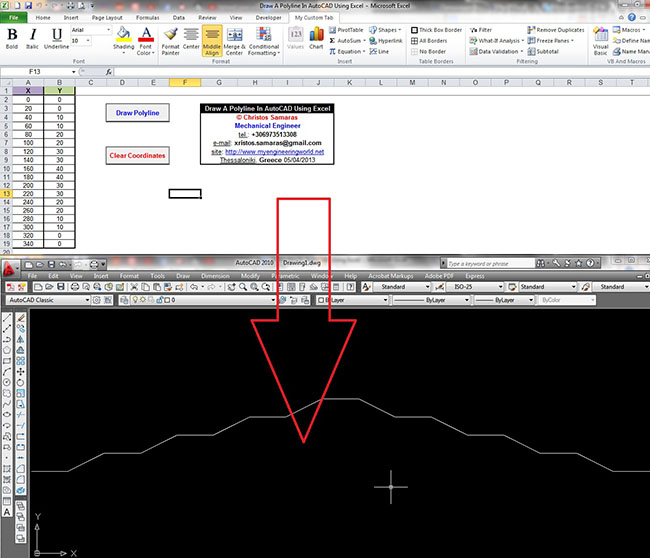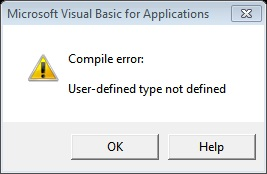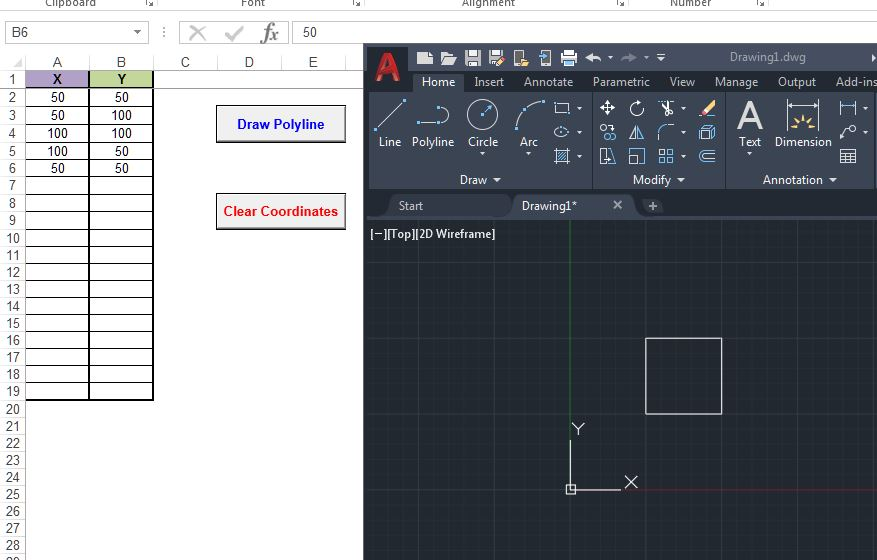# Draw A Polyline In AutoCAD Using Excel VBA

April 5, 2013### Introduction

A friend of mine, a surveying engineer, asked me recently if it is possible to draw a polyline in AutoCAD using coordinates from an Excel file. He had a large Excel file with point coordinates (x, y), and he wanted to connect them through a polyline to create a 2D profile. I manage to fulfill his request by using a lightweight polyline, a 2D line consisting of straight and arched segments.

I thought that the interaction between Excel and AutoCAD using VBA might be useful for all types of engineers using AutoCAD (primarily civil and surveying engineers), so I decided to post the VBA code here. Although the example is quite simple, it illustrates the general idea of using VBA from Excel to draw objects in AutoCAD.

### VBA code to draw a polyline in AutoCAD using early binding

``````Option Explicit
Option Private Module

Sub DrawPolyline()

'Draws a polyline in AutoCAD using X and Y coordinates from sheet Coordinates.

'By Christos Samaras
'http://www.myengineeringworld.net

'In order to use the macro you must enable the AutoCAD library from VBA editor:
'Go to Tools -> References -> Autocad 1234 Type Library, where 1234 depends

'Declaring the necessary variables.
Dim LastRow As Long
Dim dblCoordinates() As Double
Dim i As Long
Dim j As Long
Dim k As Long

shCoordinates.Activate

'Find the last row.
With shCoordinates
LastRow = .Cells(.Rows.Count, "A").End(xlUp).Row
End With

'Check if there are at least two points.
If LastRow < 3 Then
MsgBox "There not enough points to draw the polyline!", vbCritical, "Points Erro"
Exit Sub
End If

On Error Resume Next
On Error GoTo 0

'If AutoCAD is not opened create a new instance and make it visible.
End If

'Check if there is an active drawing.
On Error Resume Next
On Error GoTo 0

'No active drawing found. Create a new one.
End If

'Get the array size.
ReDim dblCoordinates(2 * (LastRow - 1) - 1)

'Pass the coordinates to array.
k = 0
For i = 2 To LastRow
For j = 1 To 2
dblCoordinates(k) = shCoordinates.Cells(i, j)
k = k + 1
Next j
Next i

'Draw the polyline either at model space or at paper space.
Else
End If

'Leave the polyline open (the last point is not connected with the first point.
'Set the next line to true if you need to connect the last point with the first one.

'Zooming in to the drawing area.

'Inform the user that the polyline was created.
MsgBox "The polyline was successfully created!", vbInformation, "Finished"

End Sub

Sub ClearCoordinates()

Dim LastRow As Long

shCoordinates.Activate

'Find the last row.
With shCoordinates
LastRow = .Cells(.Rows.Count, "A").End(xlUp).Row
.Range("A2:B" & LastRow).ClearContents
.Range("A2").Select
End With

End Sub ``````

### Demonstration video

The short video below demonstrates the results of the above VBA code.

### Note

If you try to use the above VBA code and get the following error “Compile error: User-defined type not defined” (see figure below), the first thing you should do is to check if the AutoCAD reference in the VBA editor is enabled. So, go to Tools => References => and enable Autocad 1234 Type Library, where 1234 depends on your AutoCAD version (i.e., 2010, 2011, 2012, etc.) you have installed on your computer.The above code was successfully tested in Excel and AutoCAD 2010. However, it should also work in other Excel/AutoCAD versions.

Category: Office Tips

### Christos Samaras

Hi, I am Christos, a Mechanical Engineer by profession (Ph.D.) and a Software Developer by obsession (10+ years of experience)! I founded this site back in 2011 intending to provide solutions to various engineering and programming problems.

• Hi, Robert,

In the sample workbook, you can simply enter the coordinates you need (it’s not necessary to start at 0, 0).
Check the image in the next link where I created a square starting at the point (50, 50).I hope it helps!

Best Regards,
Christos

•Robert says:

Sir how can you Start the polygon with the coordinates that you want not with the coordinates (0,0), because evertime i create a polygon it all starts at coordinate(0,0)

• Thank you very much for the interesting suggestions. I will work on the two scenarios and I will create a post based on them. From a quick thought, I think that is feasible to be done…

•JakeJimenez says:

Hi Christos, very nice code. However, as a quantity surveyor, I would really appreciate it more if you can post the reverse code of what you have posted. That is, in AutoCAD, polyline (PL command) is used to measure length, area/volume and the code writes the measurement to an open excel table, directly. Every after polyline drawn, an add-in button will extract the measurement and paste it directly on to the open excel sheet. This is the first scenario.Second scenario is, draw and name all the polylines using AutoCAD (on a separate layer i think) then using an add-in button, all the measurement data will be exported onto an open excel sheet or create one sheet if an excel sheet is not open. (I use AutoCAD 2010 with VBA and Excel 2010 with VBA as well).Thanks